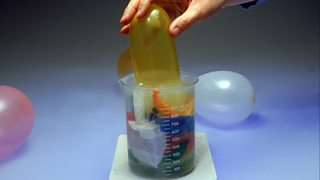# Volume and pressure in gases – the gas laws

## Boyle’s law

Decreasing the volume of a gas increases the pressure of the gas. An example of this is when a gas is trapped in a cylinder by a piston. If the piston is pushed in, the gas particles will have less room to move as the volume the gas occupies has been decreased.

As the pressure applied to a piston is doubled, the volume inside a cylinder is halved

Because the volume has decreased, the particles will collide more frequently with the walls of the container. Each time they collide with the walls they exert a on them. More collisions mean more force, so the will increase.

When the volume decreases, the pressure increases. This shows that the pressure of a gas is to its volume.

This is shown by the following equation - which is often called Boyle’s law. It is named after 17th century scientist Robert Boyle.

P1V1 = P2V2

where:

P1 is the initial pressure

V1 is the initial volume

P2 is the final pressure

V2 is the final volume

It can also be written as:

pressure1 × volume1 = pressure2 × volume2

Note that volume is measured in metres cubed (m3) and pressure in pascals (Pa).

It means that for a gas at a constant temperature, pressure × volume is also constant. So increasing pressure from pressure1 to pressure2 means that volume1 will change to volume2, providing the temperature remains constant.

Question

A sealed syringe contains 10 × 10-6 m3 of air at 1 × 105 . The plunger is pushed until the volume of trapped air is 4 × 10-6 m3. If there is no change in temperature what is the new pressure of the gas?

P1 = 1 × 105 Pa

V1 = 10 × 10-6 m3

V2 = 4 x 10-6 m3

P1V1 = P2V2

Therefore:

$p_{2} = \frac{p_{1}{V_{1}}}{V_{2}}$

$p_{2} = \frac{{1 \times 10^{5} \times 10 \times 10^{-6}}}{4 \times 10^{-6}}$

P2 = 2.5 × 105 Pa

The new pressure in the syringe is 2.5 × 105 Pa

## Charles’ law

Charles’ law describes the effect of changing temperature on the volume of a gas at constant pressure. It states that:

$volume_{1} = volume_{2} \times \frac{temperature_{1}}{temperature_{2}}$

$V_{1} = V_{2} \times \frac{T_{1}}{T_{2}}$

where:

V1 is the initial volume

V2 is the final volume

T1 is the initial temperature

T2 is the final temperature

Note that volume is measured in metres cubed (m3) and temperature in kelvin (K).

This means that if a gas is heated up and the pressure does not change, the volume will. So for a fixed mass of gas at a constant pressure, volume ÷ temperature remains the same.

The volume of a gas rises as its temperature is raisedBalloons shrink when placed inside a beaker of cold liquid nitrogen